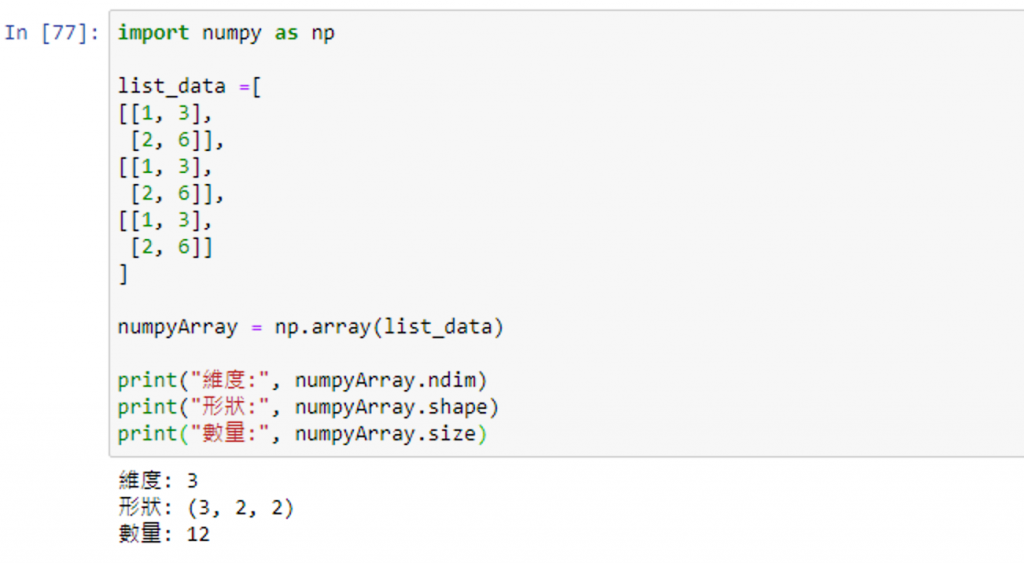#DAY 26
1
Software Development

## [2020鐵人賽Day26]糊裡糊塗Python就上手-Numpy的觀念與運用(上)

### What is Numpy?

Numpy 是 Python 資料科學計算模組，主要用於資料處理上

### 了解 Numpy 陣列

Numpy 使用多維陣列 ndarray 來取代 Python 的串列資料，是個可以裝載相同類型資料的多維容器

1 2 3

1 2 3
4 5 6

### 使用介紹

#### 使用前需要先導入 Numpy 模組(為了後續撰寫方便，可以自行取別名)

``````import numpy as np
``````

#### 建立陣列 array()

``````numpy.array(object, [其餘參數])
``````

dtype = None, order = None, subok = False, ndmin = 0

array參數 說明
object 串列或元組資料
dtype 陣列的資料型態

(還有其他參數，此篇僅說到將使用到的，若感興趣可自行查詢學習)

``````import numpy as np

v1 = np.array((1, 2, 3, 4, 5)) # 使用 list 資料型態
v2 = np.array([1.1, 2.2, 3.3, 4.4, 5.5]) # 使用 tuple 資料型態

print(v1)
print("v1 type:%s"%(type(v1))) # 印出 v1 資料型態
print(v2)
print("v2 type:%s"%(type(v2))) # 印出 v2 資料型態
``````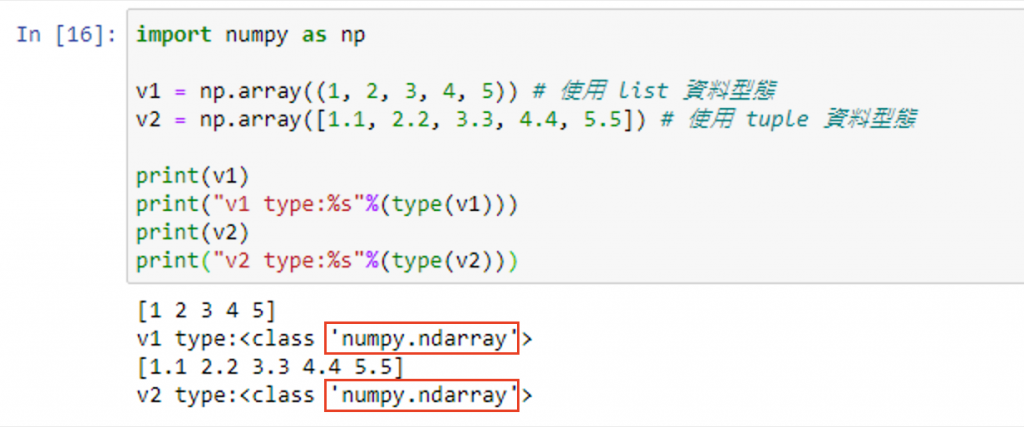#### 查詢或設定該陣列型別 dtype

``````import numpy as np

v1 = np.array((1, 2, 3, 4, 5))
v2 = np.array([1.1, 2.2, 3.3, 4.4, 5.5])
v3 = np.array([1.1, 2.2, 3.3, 4.4, 5.5], dtype=int) # 轉換資料型態為 int

print("v1.dtype:%s"%(v1.dtype)) # 印出 v1 資料型態
print("v2.dtype:%s"%(v2.dtype)) # 印出 v2 資料型態
print("v2:%s"%(v2)) # 印出 v2 資料內容
print("v3:%s"%(v3)) # 印出 v3 資料內容
print("v3.dtype:%s"%(v3.dtype)) # 印出 v3 資料型態
``````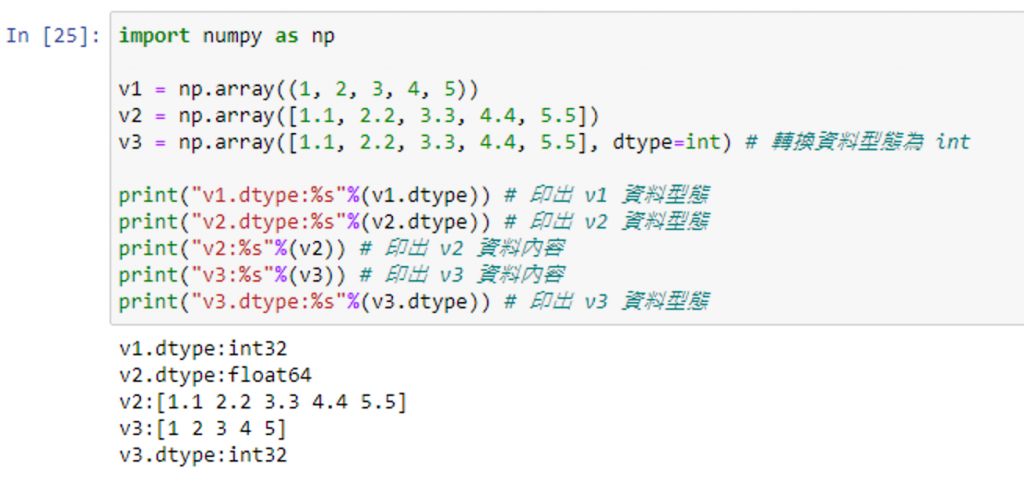#### 建立有順序的整數數列 arange()

arange 其實跟 range 有點相似，可以建立等距的整數數列

(若只有終止值，沒有起始值的話，起始值會以 0 開始)

``````numpy.arange([起始值], 終止值, [間隔值])
``````

``````import numpy as np

arangeValue = np.arange(1, 20, 3)
print(arangeValue)
``````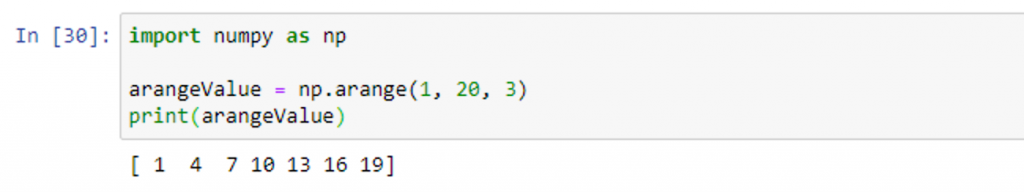#### 建立同等陣列：建立全部都是 0 的陣列 zeros()

``````numpy.zeros((陣列各維度用逗號區隔))
``````

``````import numpy as np

zeroValue = np.zeros((3))
print(zeroValue)

zeroValue2 = np.zeros((3, 5))
print(zeroValue2)
``````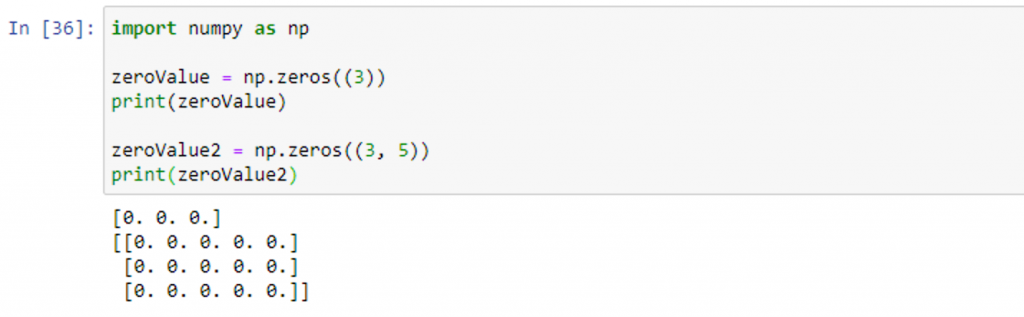#### 建立同等陣列：建立全部都是 1 的陣列 ones()

``````numpy.ones((陣列各維度用逗號區隔))
``````

``````import numpy as np

oneValue = np.ones((3))
print(oneValue)

oneValue2 = np.ones((3, 5))
print(oneValue2)
``````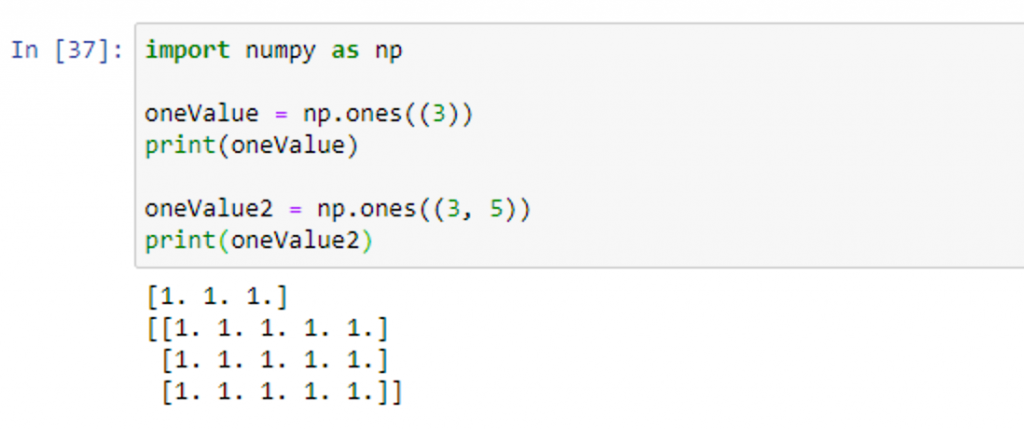#### 建立等距陣列 linspace()

``````numpy.linspace(起始值, 終止值, 元素個數)
``````

``````import numpy as np
linspaceValue = np.linspace(1, 10, 3)
print(linspaceValue)
``````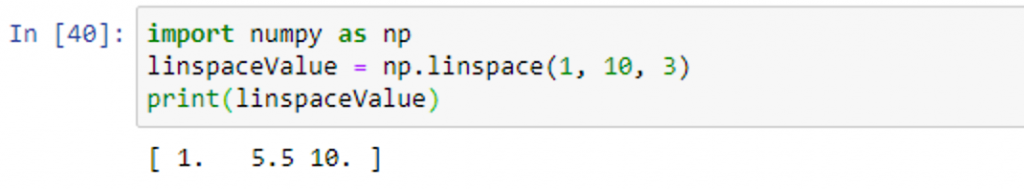#### 建立隨機值陣列 empty()

``````numpy.empty((陣列各維度用逗號區隔))
``````

``````import numpy as np
emptyValue = np.empty((2))
print(emptyValue)
``````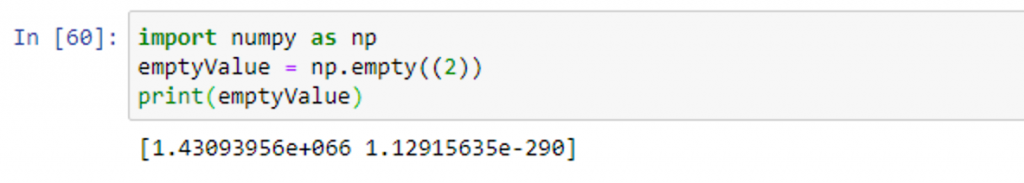### 實際體驗-建立三維陣列，並印出「維度 ndim」、「形狀 shape」、「數量 size」

ndarray 物件 說明
ndarray.ndim ndarray 物件的維度
ndarry.shape ndarry 物件的每一個維度的大小
ndarry.size ndarry 物件所組成之 array 的總元素數量，回應之數值會等於 ndarray.shape 的每個元素相乘
``````import numpy as np

list_data =[
[[1, 3],
[2, 6]],
[[1, 3],
[2, 6]],
[[1, 3],
[2, 6]]
]

numpyArray = np.array(list_data)

print("維度:", numpyArray.ndim)
print("形狀:", numpyArray.shape)
print("數量:", numpyArray.size)
``````